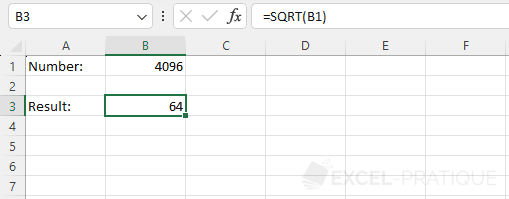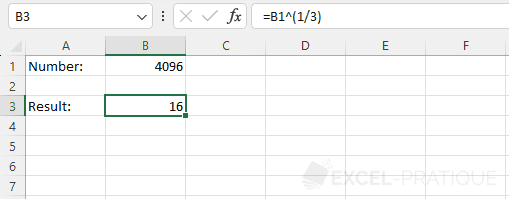# Excel Function: SQRT

The Excel function SQRT calculates the square root of a number.

Usage:

`=SQRT(number)`

## Usage example

Select the SQRT function and simply enter the number (or the cell containing the number) you want to get the square root of:

``=SQRT(B1)``## Alternative

You can also directly calculate the square root with the exponent 1 / 2 or 0.5:

``=B1^0.5``Or for example with 1 / 3 for the cubic root:

``=B1^(1/3)``